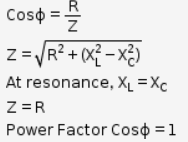# Value of power factor of L.C.R circuit which is in the state of resonance?

Value of power factor of L.C.R circuit which is in the state of resonance?• At resonance, Inductive resistance (XL) is equal to capacitive resistance (Xc)
• The Power factor is nothing but cosine of phase angle between voltage and current.
• For RLC circuit, the pf is also given by• Therefore, the value of power factor of L.C.R circuit which is in the state of resonance is unity (one).